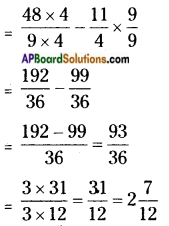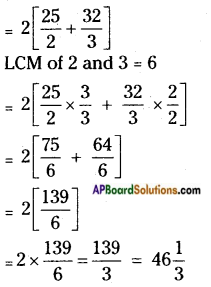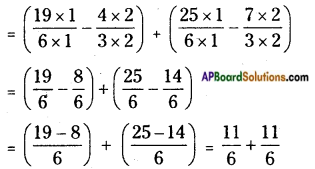# AP Board 6th Class Maths Solutions Chapter 5 Fractions and Decimals Unit Exercise

AP State Syllabus AP Board 6th Class Maths Solutions Chapter 5 Fractions and Decimals Unit Exercise Textbook Questions and Answers.

## AP State Syllabus 6th Class Maths Solutions 5th Lesson Fractions and Decimals Unit Exercise

Question 1.
The sum of two fractions is 5$$\frac{3}{9}$$. If one fraction is 2$$\frac{3}{4}$$, then find the other fraction.
Given one fraction = 2$$\frac{3}{4}$$ = $$\frac{11}{4}$$
Sum of two fractions = 5$$\frac{3}{9}$$ = $$\frac{48}{9}$$
Now, $$\frac{11}{4}$$ + second fraction = $$\frac{48}{9}$$
Second fraction = $$\frac{48}{9}$$ – $$\frac{11}{4}$$
LCM of 9 and 4 is 36Question 2.
A rectangle sheet of paper is of length 12$$\frac{1}{2}$$ and breadth 10$$\frac{2}{3}$$. Find its perimeter.
Length of rectangular sheet = l = 12$$\frac{1}{2}$$ = $$\frac{25}{2}$$ m
Breadth of rectangular sheet = b = 10$$\frac{2}{3}$$ = $$\frac{32}{3}$$ m
Perimeter of rectangular sheet = 2(1 + b)Question 3.
Simplify: $$\left(3 \frac{1}{6}-1 \frac{1}{3}\right)+\left(4 \frac{1}{6}-2 \frac{1}{3}\right)$$
$$\left(3 \frac{1}{6}-1 \frac{1}{3}\right)+\left(4 \frac{1}{6}-2 \frac{1}{3}\right)$$
First convert the given mixed fractions into improper fractions.
$$\left(\frac{19}{6}-\frac{4}{3}\right)$$ + $$\left(\frac{25}{6}-\frac{7}{3}\right)$$
Then subtract the fractions which are in brackets by making them like fractions.Now add the fractions = $$\frac{11+11}{6}$$ = $$\frac{22}{6}$$ = 3$$\frac{4}{6}$$Question 4.
By what number should 3$$\frac{1}{16}$$ be multiplied to get 9$$\frac{3}{16}$$?
Given fraction = 3$$\frac{1}{16}$$ = $$\frac{49}{16}$$
Product = 9$$\frac{3}{16}$$ = $$\frac{147}{16}$$
Fraction × fraction to be multiplied = $$\frac{147}{16}$$
$$\frac{49}{16}$$ × other number = $$\frac{147}{16}$$
other number = $$\frac{147}{16}$$ ÷ $$\frac{49}{16}$$ = $$\frac{147}{16}$$ × $$\frac{16}{49}$$
Other number = 3
∴ Number to be multiplied = 3.

Question 5.
The length of the staircase is 5$$\frac{1}{2}$$ m. If one step is set at $$\frac{1}{4}$$ m. then, how many steps will be there in the staircase?
Given length of staircase = 5$$\frac{1}{2}$$ m = $$\frac{11}{2}$$ m
Length of each step = $$\frac{1}{4}$$ m
Length of each step × number of steps = length of staircase
$$\frac{1}{4}$$ × Number of steps = $$\frac{11}{2}$$
Number of steps = $$\frac{11}{2}$$ ÷ $$\frac{1}{4}$$ = $$\frac{11}{2}$$ × $$\frac{4}{1}$$ = 11 × 2 = 22
∴ Number of steps in the staircase = 22Question 6.
Simplify: 23.5 – 27 + 35.4 – 17
Given 23.5 – 27 + 35.4 – 17
First make them like decimals.
= 23.5 – 27.0 + 35.4 – 17.0
= (+23.5 + 35.4) + (-27.0 – 17.0)
= 58.9 + (-44.0)
= 58.9 – 44.0
= 14.9

Question 7.
Sailaja bought 3.350kg of potatoes, 2.250kg of tomatoes and some onions. If the weight of the total items are 10.250 kg. Then, find the weight of onions.
Weight of potatoes = 3.350 kg
Weight of tomatoes = 2.250 kg
Weight of total items = 10.250 kg
Weight of onions = ?
Weight of (potatoes + tomatoes + onions) = 10.250 kg
(3.350 + 2.250) + onions weight = 10.250
5.600 + onions weight = 10.250
Onions weight = 10.250 – 5.600
∴ Weight of onions = 4.650 kg.Question 8.
What should be subtracted from 7.1 to get 0.713?